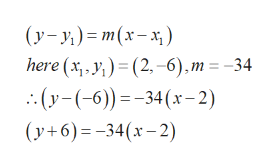# What is the equation, in point-slope form, for a line that goes through ​ (2, −6) ​ and has a slope of ​ −34 ​ ?  y+6=−34(x−2)y−6=−34(x+2)y+6=−34(x+2)y−6=34(x−2)

Question
8 views

What is the equation, in point-slope form, for a line that goes through ​ (2, −6) ​ and has a slope of ​ −34 ​ ?

• y+6=−34(x−2)
• y−6=−34(x+2)
• y+6=−34(x+2)
• y−6=34(x−2)
check_circle

Step 1

Given,

The line passing through the point ​ (2, −6)  and the slope is -34

Step 2

Therefore, the equation of the line passing through the p...help_outlineImage Transcriptionclose(y- y;) = m(x- x; ) here (x, , y;) = (2,-6),m = -34 .:(y-(-6)) =-34(x – 2) (y+6)=-34(x- 2) fullscreen

### Want to see the full answer?

See Solution

#### Want to see this answer and more?

Solutions are written by subject experts who are available 24/7. Questions are typically answered within 1 hour.*

See Solution
*Response times may vary by subject and question.
Tagged in

### Other